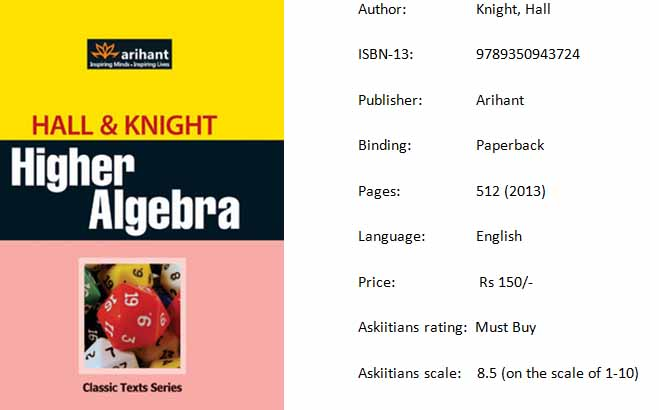# Hall and Knight Algebra is one of the best books for JEE preparation!Algebra by Hall and Knight is one of the best books for JEE preparation. Students preparing for IIT JEE and other engineering entrance exams as well as students appearing for board exams should read this everyday, especially to master Algebra and Probability. Hall and Knight have explained the concepts logically in their book.

The book is quite interesting and challenging. Authored by some of the best authors, Hall and Knight Algebra book is quite affordable too.

## Topics covered in the book are:

• Ratio

• Proportion

• Variation

• Arithmetical Progression

• Geometrical Progression

• Harmonical Progression Theorems Connected with The Progression

• Scales of Notation

• Surds and Imaginary Quantities

• The Theory of Quadratic Equations

• Miscellaneous Equations

• Permutations and Combinations

• Mathematical Induction

• Binomial Theorem Positive Integral Index

• Binomial Theorem. Any Index

• Multinomial Theorem

• Logarithms

• Exponential and Logarithmic Series

• Interest and Annuities

• Inequalities

• Limiting Values and Vanishing Fractions

• Convergency and Divergency of Series

• Undetermined Coefficients

• Partial Fractions

• Recurring Series

• Continued Fractions

• Indeterminate Equations of the First Degree

• Recurring Continued Fractions

• Indeterminate Equations of the Second Degree

• Summation of Series

• Theory of Numbers

• The General Theory of Continued Fractions

• Probability

• Chapter 33 Determinants

• Chapter 34 Miscellaneous Theorems and Examples

• Chapter 35 Theory of Equations

Hall and Knight Algebra includes lots of solved and unsolved examples, which offer excellent practice to students preparing for competitive exams. Solutions to the problems in the Higher Algebra by Hall and Knight are arranged in a point-wise manner with step-by-step explanations, making them easier to understand.

Books such as pre-algebra book, Higher School Mathematics by Hall and Knight, and Hall and Knight Algebra book are interlinked and hence, it is difficult to classify them as Class 11 or Class 12 books.

Many of the sub-topics that JEE aspirants need to master are missing in the book like position of roots, range of quadratic functions, and rational inequalities. Besides, the book follows a subjective pattern, not best suitable for JEE preparation. It is best for students of Class IX and X to develop an interest in Algebra as it introduces them to several new theorems in a very interesting manner.

Other Algebra books you may want to buy are:

• Arihant

• Barnard and Child

• K.P. Basu